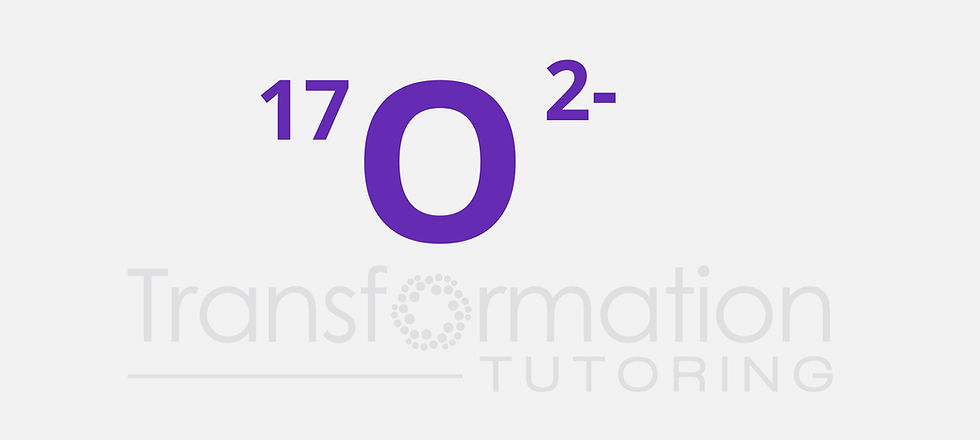top of page
Search

# Write the symbol for an ion with 8 protons, 9 neutrons, and 10 electrons

Write the symbol for an ion with 8 protons, 9 neutrons, and 10 electrons:

Number of protons is equal to the atomic number and is unique for each element. Looking at the Periodic table, we can see that oxygen has an atomic number of 8. Mass number is the sum of protons and neutrons and is equal to 8+9=17.

Each proton has a +1 charge and each electron has a -1 charge. We get +8-10= -2 as the charge for the ion.Write the symbol for an ion with 8 protons, 9 neutrons, and 10 electrons

Learn Organic Chemistry HERE

Get an online Organic Chemistry tutor HERE